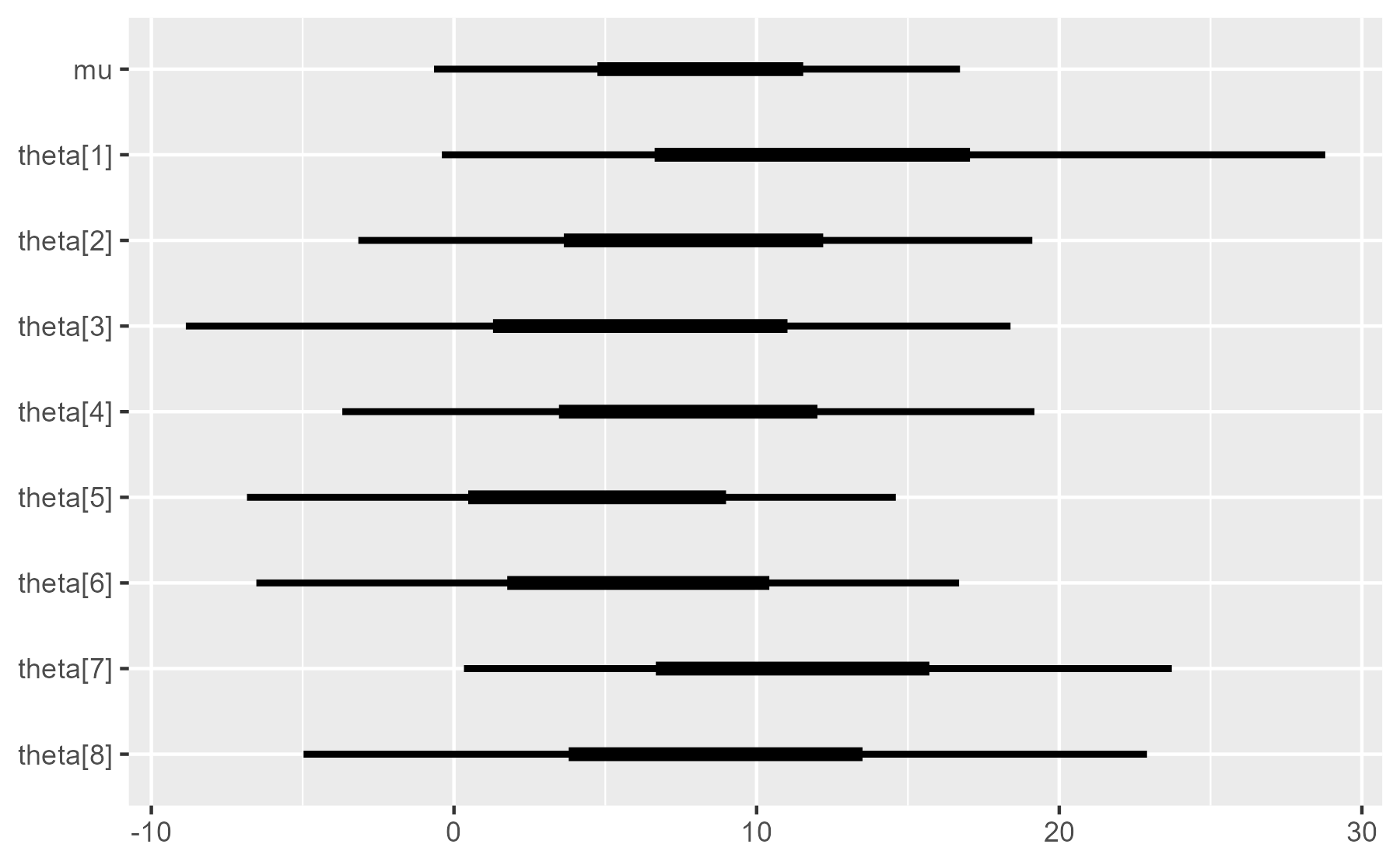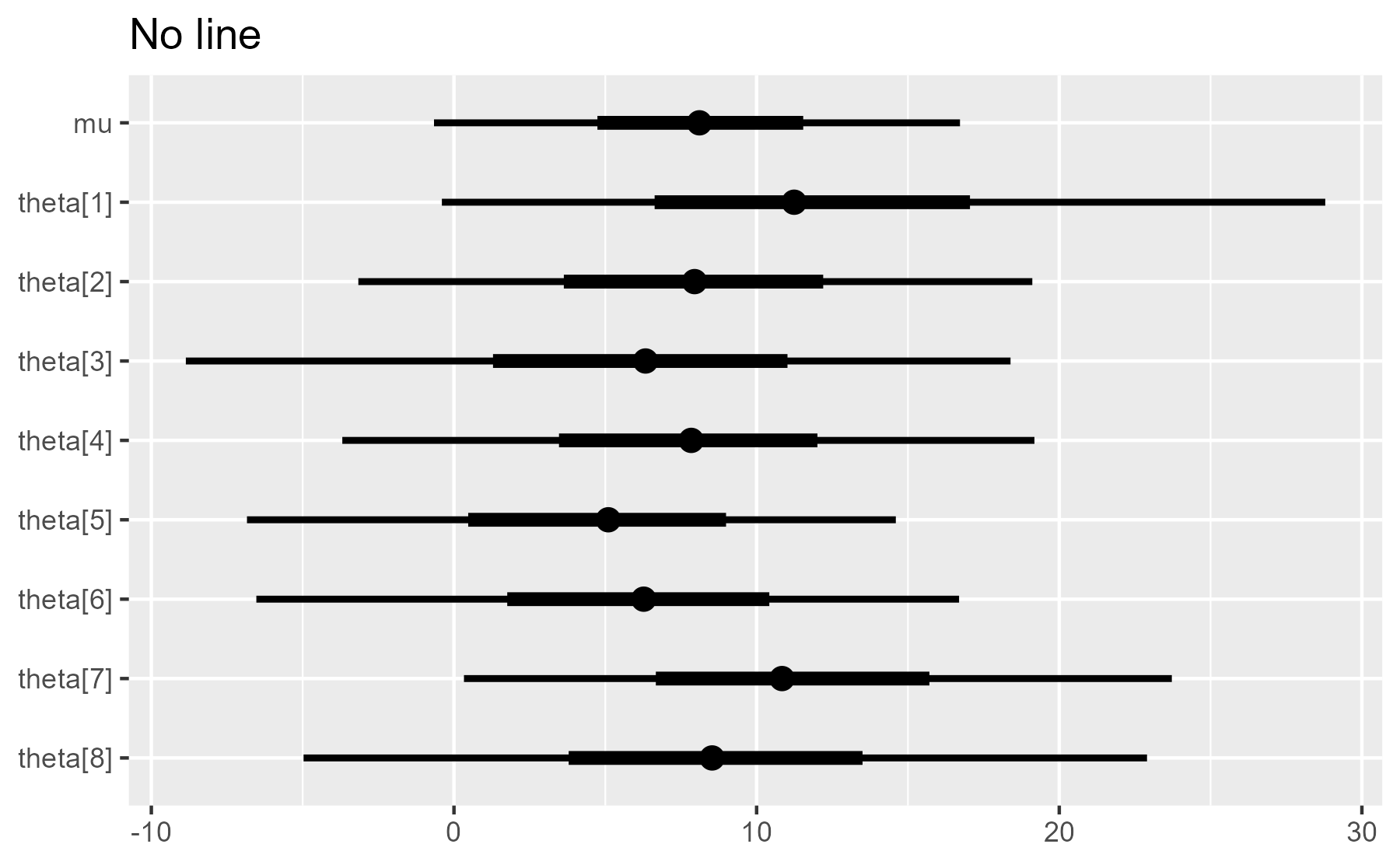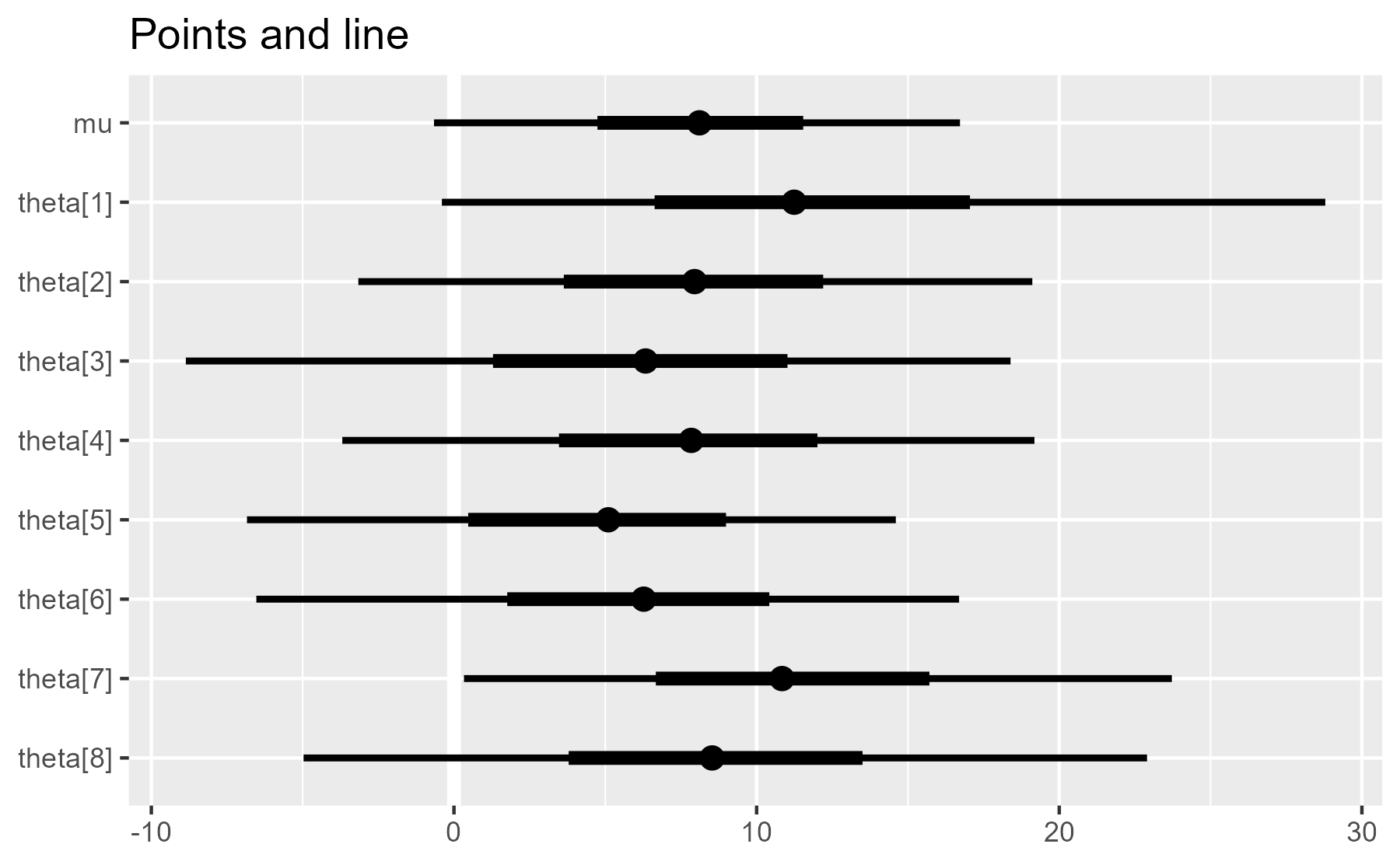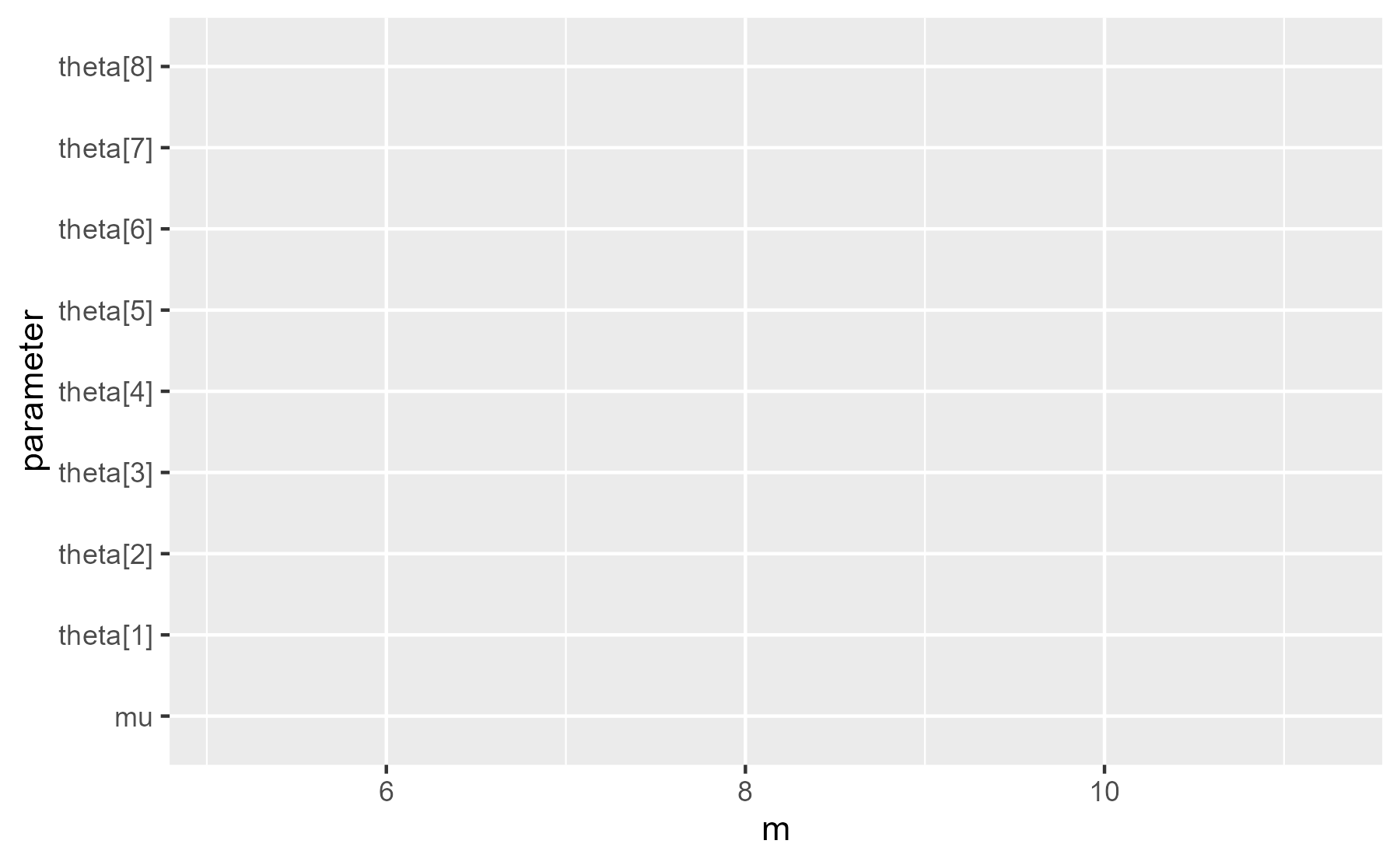Want to share your content on R-bloggers? click here if you have a blog, or here if you don't.

Recently, I joined the development team for bayesplot, an R package by the Stan team for plotting Bayesian models. Because visualizing Bayesian models in ggplot2 is a recurring topic here, it was a natural fit. So from time to time, I’ll post about some programming techniques and new features we develop in the bayesplot package.

For this post, I describe one of strategies I have been using to clean up and simplify some of the plotting code in the package: avoid if-branches by sometimes plotting nothing.

## Warm-up example

Let’s start with a non-plotting example. We consider a function that takes a list of counts of people and returns the total number of people. That sum, however, is controlled by some of the function’s arguments:

```head_count <- function(data, staff = FALSE, faculty = FALSE) {
total <- data[["students"]]

if (staff) {
total <- total + data[["staff"]]
}

if (faculty) {
total <- total + data[["faculty"]]
}

total
}

data <- list(students = 10, staff = 3, faculty = 2)
#>  10
#>  13
#>  12
```

The function as it’s written works fine… but the if-branches get in the way. The main job of the function is to assemble something (a sum) from pieces of data, but that assembly is split across the if-branches. The `total` is updated in-place twice (`total <- total + ...`). I would rather combine all the data in one fell swoop.

One way to move the data-assembly out of these if-branches is to make the data conditional. That is, instead of doing the addition in the `if` statements, we do all the addition at once but just add 0 when a piece of data isn’t needed. This technique simplifies the function definition:

```head_count <- function(data, staff = FALSE, faculty = FALSE) {
staff <- if (staff) data[["staff"]] else 0
faculty <- if (faculty) data[["faculty"]] else 0

data[["students"]] + staff + faculty
}

#>  10
#>  13
#>  12
```

For operations where we combine things to get a new thing, there’s often an identity element: a value we can plug in as a placeholder value without changing the results. Some examples:

```# multiplication
pi * 1
#>  3.141593

# string concatenation
paste0("a", "")
#>  "a"

# combining things
c(1, 2, 3, c())
#>  1 2 3

# data-frame binding
#>   Sepal.Length Sepal.Width Petal.Length Petal.Width Species
#> 1          5.1         3.5          1.4         0.2  setosa
#> 2          4.9         3.0          1.4         0.2  setosa
#> 3          4.7         3.2          1.3         0.2  setosa
#> 4          4.6         3.1          1.5         0.2  setosa
#> 5          5.0         3.6          1.4         0.2  setosa
#> 6          5.4         3.9          1.7         0.4  setosa

# magrittr pipes
f <- function(x, ...) x
1:2 %>% f()
#>  1 2
```

Below, I demonstrate two examples of how I applied a similar strategy in bayesplot. In ggplot2, we assemble plots by adding layers and options to a plot by stringing together statements with `+`. To handle conditional plotting elements and construct the plot all in one pass, I took advantage of ways to adding nothing to a ggplot2 plot.1

## Posterior interval plots

Here are some posterior samples for a Bayesian model, in this case the “eight schools” meta-analysis example. We want to create a plot that shows the 90% and 50% uncertainty intervals for each parameter.

We first use a helper function to clean up the data to prepare it for plotting. (At the time of writing, `mcmc_intervals_data()` is not yet in the CRAN version of bayesplot.)

```library(dplyr, warn.conflicts = FALSE)
library(bayesplot)
#> This is bayesplot version 1.4.0.9000
#> - Plotting theme set to bayesplot::theme_default()
#> - Online documentation at mc-stan.org/bayesplot
library(ggplot2)
theme_set(theme_grey())

interval_data <- shinystan::[email protected]_sample %>%
mcmc_intervals_data(pars = "mu", regex_pars = "theta") %>%
mutate_if(is.numeric, round, 2)

interval_data
#> # A tibble: 9 x 9
#>   parameter outer_width inner_width point_est    ll     l     m     h
#>      <fctr>       <dbl>       <dbl>     <chr> <dbl> <dbl> <dbl> <dbl>
#> 1        mu         0.9         0.5    median -0.66  4.74  8.11 11.54
#> 2  theta         0.9         0.5    median -0.40  6.63 11.24 17.05
#> 3  theta         0.9         0.5    median -3.16  3.63  7.95 12.20
#> 4  theta         0.9         0.5    median -8.86  1.29  6.33 11.02
#> 5  theta         0.9         0.5    median -3.69  3.47  7.84 12.01
#> 6  theta         0.9         0.5    median -6.84  0.47  5.10  8.99
#> 7  theta         0.9         0.5    median -6.53  1.76  6.27 10.42
#> 8  theta         0.9         0.5    median  0.33  6.67 10.84 15.71
#> 9  theta         0.9         0.5    median -4.97  3.79  8.53 13.50
#> # ... with 1 more variables: hh <dbl>
```

Below is the basic plot we want to create. (Normally, in bayesplot, we would use `mcmc_intervals()` to make this plot, but for the sake of illustration, we will write a version of it from scratch.

```ggplot(interval_data) +
aes_(y = ~ parameter, yend = ~ parameter) +
geom_segment(aes_(x = ~ ll, xend = ~ hh), size = 1) +
geom_segment(aes_(x = ~ l, xend = ~ h), size = 2) +
scale_y_discrete(limits = rev(interval_data\$parameter)) +
labs(x = NULL, y = NULL)
```Wait, what’s going on with the tildes? We use `aes_(y = ~ parameter)` because in an R package, writing `aes(y = parameter)` would raise an undefined global variable warning :warning: during CRAN package checks. The code looks like it uses a variable called `parameter`, but a variable with that name has not been defined in the code yet. The formula form `~ parameter` “quotes” the variable name, so it doesn’t appear as a global variable.

We are going to wrap this code in an R function along with two annotation options:

1. whether to draw point estimates (medians) over the intervals
2. whether to draw a vertical reference line at x = 0

Here is how we might write the function with branching code.

```plot_intervals <- function(data, draw_points = TRUE, draw_ref_line = TRUE,
line_position = 0) {
p <- ggplot(data) +
aes_(y = ~ parameter, yend = ~ parameter)

if (draw_ref_line) {
p <- p +
geom_vline(xintercept = line_position, size = 2, color = "white")
}

p <- p +
geom_segment(aes_(x = ~ ll, xend = ~ hh), size = 1) +
geom_segment(aes_(x = ~ l, xend = ~ h), size = 2)

if (draw_points) {
p <- p + geom_point(aes_(x = ~ m), size = 3)
}

p +
scale_y_discrete(limits = rev(data\$parameter)) +
labs(x = NULL, y = NULL)
}

# Test the code
plot_intervals(interval_data) + ggtitle("Points and line")
plot_intervals(interval_data, draw_points = FALSE) + ggtitle("No points")
plot_intervals(interval_data, draw_ref_line = FALSE) + ggtitle("No line")
```As we can see, the plot is built up incrementally throughout the function. The plot object `p` is updated three times (the `p <- p + ...` parts). This makes it more difficult to understand the function when reading it and increases the chance that we might do something wrong when working on this code.

We can improve the readability by using empty or placeholder elements to move the plot updates outside of the if-branches.

## Do nothing by plotting an empty dataframe

ggplot2 is built to work on dataframe columns, and if there is no data in a column, it will quietly plot nothing. Therefore, one way to do nothing is to run the normal `geom_` plotting function but on an empty dataframe. Here, we apply that strategy for the point estimates by toggling between the original data or zero-row alternative in the first line.

```plot_intervals <- function(data, draw_points = TRUE, draw_ref_line = TRUE,
line_position = 0) {
maybe_points <- if (draw_points) data else data[0, ]

p <- ggplot(data) +
aes_(y = ~ parameter, yend = ~ parameter)

if (draw_ref_line) {
p <- p +
geom_vline(xintercept = line_position, size = 2, color = "white")
}

p +
geom_segment(aes_(x = ~ ll, xend = ~ hh), size = 1) +
geom_segment(aes_(x = ~ l, xend = ~ h), size = 2) +
geom_point(aes_(x = ~ m), data = maybe_points, size = 3) +
scale_y_discrete(limits = rev(data\$parameter)) +
labs(x = NULL, y = NULL)
}

# Test the code
plot_intervals(interval_data) + ggtitle("Points and line")
plot_intervals(interval_data, draw_points = FALSE) + ggtitle("No points")
```This is a marked improvement over the original, as most of the plot construction happens at the end of the function.

## Do nothing with `geom_blank`

Another way to go nothing is to add `geom_blank()`. In fact, this is what ggplot2 does when we print a ggplot2 object without any data.

```ggplot(interval_data) +
aes(x = m, y = parameter)
```When we inspect the last plot that was displayed, we see `geom_blank()` is one of the layers:

```last_plot()[["layers"]]
#> []
#> geom_blank: na.rm = FALSE
#> stat_identity: na.rm = FALSE
#> position_identity
```

As a first pass for technique, we toggle between the plotting functions `geom_vline()` and `geom_blank()`. This version simplifies the plot construction to a single stream of plot additions.

```plot_intervals <- function(data, draw_points = TRUE, draw_ref_line = TRUE,
line_position = 0) {
maybe_points <- if (draw_points) data else data[0, ]
geom_maybe_vline <- if (draw_ref_line) geom_vline else geom_blank

ggplot(data) +
aes_(y = ~ parameter, yend = ~ parameter) +
geom_maybe_vline(xintercept = line_position, size = 2, color = "white") +
geom_segment(aes_(x = ~ ll, xend = ~ hh), size = 1) +
geom_segment(aes_(x = ~ l, xend = ~ h), size = 2) +
geom_point(aes_(x = ~ m), data = maybe_points, size = 3) +
scale_y_discrete(limits = rev(data\$parameter)) +
labs(x = NULL, y = NULL)
}

# Test the code
plot_intervals(interval_data) + ggtitle("Points and line")
plot_intervals(interval_data, draw_ref_line = FALSE) + ggtitle("No line")
#> Warning: Ignoring unknown parameters: xintercept, size, colour
```But it also issues a warning when it toggles to `geom_blank()`. That might alarm some users, so we need to fix that. For bayesplot, I wrote a helper called `geom_ignore()` as a version of `geom_blank()` that ignores any input arguments. The function recieves any number of arguments in the `...` placeholder argument, but the function does nothing with those dots. It just ignores them.

```geom_ignore <- function(...) {
geom_blank(mapping = NULL, data = NULL,
show.legend = FALSE, inherit.aes = FALSE)
}
```

For completeness, here is the final form using `geom_ignore()`.

```plot_intervals <- function(data, draw_points = TRUE, draw_ref_line = TRUE,
line_position = 0) {
maybe_points <- if (draw_points) data else data[0, ]
geom_maybe_vline <- if (draw_ref_line) geom_vline else geom_ignore

ggplot(data) +
aes_(y = ~ parameter, yend = ~ parameter) +
geom_maybe_vline(xintercept = line_position, size = 2, color = "white") +
geom_segment(aes_(x = ~ ll, xend = ~ hh), size = 1) +
geom_segment(aes_(x = ~ l, xend = ~ h), size = 2) +
geom_point(aes_(x = ~ m), data = maybe_points, size = 3) +
scale_y_discrete(limits = rev(data\$parameter)) +
labs(x = NULL, y = NULL)
}

# Test the code
plot_intervals(interval_data) + ggtitle("Points and line")
plot_intervals(interval_data, draw_ref_line = FALSE) + ggtitle("No line")
```### A final note on `NULL` and why I avoid it

I should come clean and note that there is not really an identity element for ggplot2 construction. Unlike adding 0 to a number, using these techniques to add nothing to a plot still updates the plot object. For the plot object below, the first layer says `geom_blank` and the last layer says `geom_point`. Visually, we have done nothing, but internally, we left a trace behind.

```p <- plot_intervals(
interval_data,
draw_points = FALSE,
draw_ref_line = FALSE)

p[["layers"]][]
#> geom_blank: na.rm = FALSE
#> stat_identity: na.rm = FALSE
#> position_identity

p[["layers"]][]
#> mapping: x = m
#> geom_point: na.rm = FALSE
#> stat_identity: na.rm = FALSE
#> position_identity
```

After some playing around, I discovered that adding `NULL` to a ggplot doesn’t change it (e.g., `p + NULL` does not leave a trace), but I plan to avoid this technique. First, it seems like an edge-case: We are normally not supposed to add `NULL` to things, so we’re asking for trouble. (In contrast, we are supposed to add `geom_blank()` to things, so no problem there.) Second, it doesn’t seem future-proof. We might imagine a later stricter version of ggplot2 where adding `NULL` raises an error. Then all the code that relies on this one weird trick will break.

1. This technique of using an empty element with combiner functions was inspired by the concept of monoids which come up in functional programming. Monoids have a strict formal definition, and ggplot2 are certainly not monoids because everything must be added onto an initial `ggplot()` object.